# Phosphorus trifluoride (PF3) lewis dot structure, molecular geometry, electron geometry, polar or nonpolar, bond angle

Home  > Chemistry Article > PF3 lewis structure and its molecular geometry/shape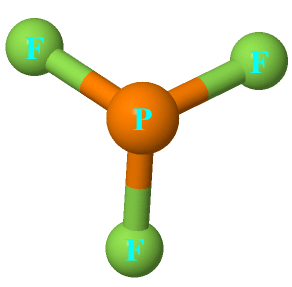Phosphorus trifluoride appears as a colorless gas and it is highly toxic in nature similar to carbon monoxide or comparable to phosgene. It is odorless, nucleophile, and weak base in nature and has a chemical formula of PF3.

In this article, we will discuss Phosphorous trifluoride (PF3) lewis dot structure, molecular geometry, electron geometry, hybridization, polar or nonpolar, its bond angle, etc.

“Phosphorus trifluoride is similar to carbon monoxide in that it is a gas which strongly binds to iron in hemoglobin, preventing the blood from absorbing oxygen.”

Properties of Phosphorous trifluoride

• It has a molar mass of 87.96 g/mol.
• It has a boiling point of −101.8 °C and a melting point of −151.5 °C.
• It has a dipole moment of 1.03 D.
• It is non-flammable gas.
• It is a highly toxic gas and reacts slowly with water
 Name of Molecule Phosphorous trifluoride Chemical formula PF3 Molecular geometry/shape of PF3 Trigonal pyramid Electron geometry of PF3 Tetrahedral Hybridization Sp³ Bond angle 96° Total Valence electron for PF3 26 The formal charge of PF3 0
Page Contents

## How to draw lewis structure of PF3

PF3 lewis structure is made up of one phosphorous atom that located at the central position and three fluorine atom that spread around the central atom. There are a total of 10 lone pairs(including central and terminal atoms) and three bonded pairs present in the lewis dot structure of PF3.

Let’s see how to draw the lewis structure of PF3 by following some simple steps-

## Simple steps for drawing the Lewis dot structure for PF3

1. Count total valence electron in PF3

In the first step, we have to find how many valence electrons are there in PF3, so that we can distribute them around central and terminal atoms with the goal of completing their octet shell.

You have two ways to find out the valence electron for a particular atom, either by looking at their periodic group or by writing their electronic configuration. We will use the method of the periodic group for finding the valence electron in PF3.

Phosphorous atom belongs to Group 5A or 15A in the periodic table, hence, it has a 5 valence electron in its outermost shell whereas fluorine atom belongs to Group 7A or 17A, hence, it has a 7 valence electron in its outermost shell.

⇒ Total valence electron in Phosphorous = 5

⇒ Total valence electron in Fluorine = 7

∴ Total valence electron available for drawing the PF3 lewis structure = 5 + 7*3 = 26 valence electrons     [∴PF3 has three fluorine atom and one phosphorous]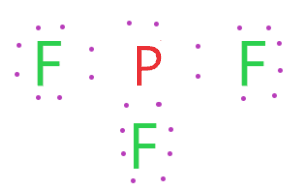2. Find the least electronegative atom and placed it at center

Which is more electronegative, phosphorous or fluorine atom? Of course, the fluorine atom(3.98) is more electronegative than phosphorous(2.19). Hence, the fluorine atom goes outside in the lewis diagram whereas the phosphorus atom fetched the seat of the central position.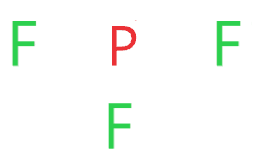3. Connect phosphorous and fluorine with a single bond

In this step, we start drawing the PF3 skeletal structure by bonding together Phosphorous and Fluorine with a single bond.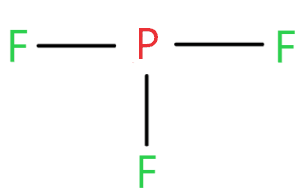Now look at this structure and count how many electrons we used till now and how many are left.

As three single bonds are used in the above structure that contains 6 electrons and we have a total of 26 valence electrons are available for drawing the PF3 lewis structure.

∴ (26 – 6) = 20 valence electrons

So, we are left with 20 valence electrons.

4. Placed remaining valence electrons starting from outer atom first

In this step, we have to place the remaining valence electrons starting from the more electronegative atom or from the outer atoms.

So, fluorine is more electronegative than phosphorous and it needs 8 electrons to complete its octet rule.

Fluorine atoms already sharing 2 electrons with the help of a single bond so it only needs 6 more electrons to complete its octet rule.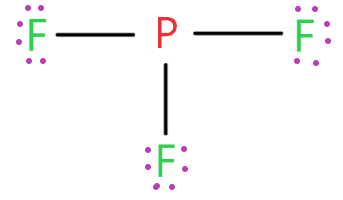As you see in the above structure, each fluorine has 6 electrons represented as dots and 2 electrons in form of a single bond, hence, the fluorine atom has a total of 8 electrons and it completed its octet comfortably.

Now find how many valence electrons are left.

Three fluorine atoms in the above structure used 18 electrons(represented as dots) from a total of 20 remaining valence electrons.

∴ (20 – 18) = 2 valence electrons

So, we left with only 2 electrons.

5. Complete central atom octet  and use covalent bond if necessary

We are left with only 2 valence electrons and by looking at the 4th step structure, we see, the central atom phosphorus has only 6 electrons(three single bonds), hence, it needs 2 more electrons to complete the octet and attains stability.

So, put the remaining two electrons on the phosphorous atom and complete its octet.### Lewis dot structure for PF3

As you see in this PF3 lewis dot structure, phosphorous and fluorine completed their octet, and everything looks fine, but, for the sake of satisfaction, we should also determine the formal charge in the above structure to know whether it is stable or not.

6. Check the stability with the help of a formal charge concept

To calculate the formal charge in the PF3 lewis structure. Use the formula given below-Let’s start with the central atom which is phosphorous in the PF3 molecule.

For phosphorous atom:

⇒ Valence electrons of phosphorous = 5

⇒ Lone pair electrons on phosphorous = 2

⇒ Bonding electrons of phosphorous(3 single bonds) = 6

∴ (5 – 2 – 6/2) = 0 formal charge on the phosphorous central atom.

Each fluorine atom in the PF3 lewis structure has the same number of lone pair and bonded pair electrons, hence, just count formal charge for just one fluorine atom

For fluorine atom

⇒ Valence electrons of fluorine = 7

⇒ Lone pair electrons on fluorine = 6

⇒ Bonding electrons around fluorine (1 single bond) = 2

∴ (7 – 6 – 2/2) = o formal charge on each fluorine atom.

Hence, each atom in PF3 gets a formal charge equal to zero.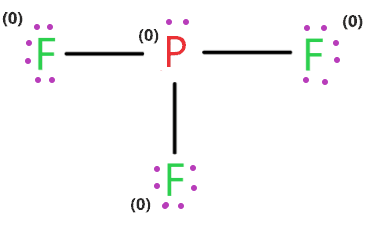Therefore, the above lewis structure of PF3 (phosphorous trifluoride) is the most stable and reliable

## What are the electron and molecular geometry of PF3?

The molecular geometry of PF3 is a trigonal pyramid and its electron geometry is tetrahedral because phosphorous has Sp³ hybridization with 5 valence electrons in its valence shell and it makes three bond pairs, one with each fluorine atom.

And one lone pair is present on the central phosphorous atom that tries to repel the bonded pairs of electrons, as a result, the adjacent atoms push apart giving its geometry trigonal pyramid.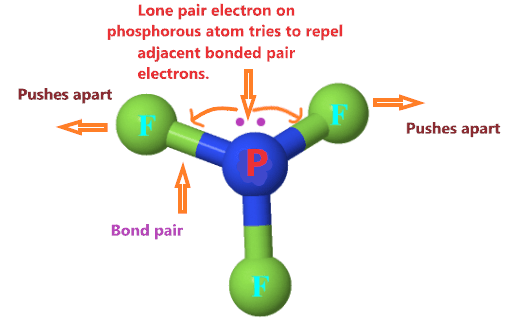As you see in the above structure, the lone pair on the phosphorus atom repel the adjacent electron pairs, as a result, the two fluorine atom in equatorial position pushes far apart giving the molecular geometry of PF3 same as a trigonal pyramid.

Let’s see how to find the molecular and electron geometry of PF3 using the VSEPR chart and AXN notation.

AXN method is a famous way of determining the molecular and electron geometry using the VSEPR chart.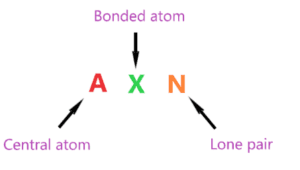AXN notation for PF3 molecule:

• A denotes the central atom, so, in the PF3 molecule, phosphorous is the central atom. A = Phosphorous
• X denotes the bonded atoms to the central atom, as we know, phosphorous is bonded with three fluorine atoms. Therefore, X = 3
• N denotes the lone pair on the central atom, as per PF3 lewis structure, phosphorous has only one lone pair. Hence, N = 1

So, the AXN notation for the PF3 molecule becomes AX3N1

As per the VSEPR chart, if a molecule central atom is attached with three bonded atoms and has contained only one lone pair on the central atom then the molecular geometry of that molecule is the trigonal pyramid in nature, and electron geometry is tetrahedral.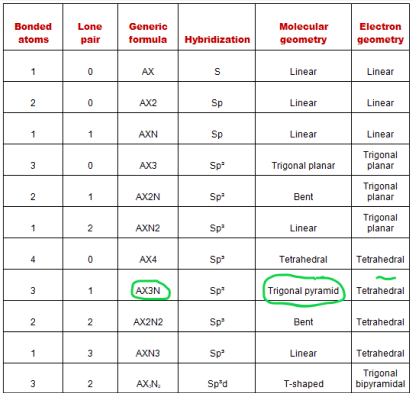Hence, the molecular shape for PF3 is a trigonal pyramid and its electron geometry is tetrahedral.## Hybridization of PF3

Let’s find the hybridization of PF3 through the steric number of its central atom.

“Steric number is the addition of a total number of bonded atoms around a central atom and the lone pair present on it.”

∴ Steric number of PF3 = (Number of bonded atoms attached to phosphorous + Lone pair on phosphorous)

As per the lewis structure of PF3, the central phosphorous atom is bonded with three fluorine atoms and it contains one lone pair also.

∴ Steric number of PF3 = (3 + 1) = 4

 Steric number Hybridization 1 S 2 Sp 3 Sp² 4 Sp³ 5 Sp³d 6 Sp³d²

So, for a steric number of four, we get the Sp3 hybridization on a phosphorous atom in the PF3 molecule.

The newly produced hybrid orbitals in PF3 molecule have 25% behavior of the s orbital and 75% behavior of the p orbital.

## The bond angle of PF3

“Phosphorus trifluoride has an F−P−F bond angle of approximately 96°“.## Phosphorous trifluoride polarity: is PF3 polar or nonpolar

PF3 is a polar molecule because of its asymmetric shape and electronegativity difference between phosphorous and fluorine which cause non-zero dipole moment.

For knowing, is PF3 polar or non-polar? we will discuss three factors 1. electronegativity 2. dipole moment 3. Geometrical or molecular shape.

## Three factors that indicate the polarity of PF3

1. Electronegativity:

The difference between the electronegativity of Phosphorous and fluorine is directly proportional to the Polarity of the PF3 molecule.

The electronegativity of the fluorine atom is 3.98 and for the phosphorous atom, its value is 2.19.

So, being more electronegativity of fluorine help it to attract more bonded electron than phosphorous. Therefore, fluorine gains a partial negative charge, and phosphorous gains a partial positive charge.

Positive and negative charges cause non-uniform charge distribution around the PF3 structure. Hence, it also causes PF3 to become polar in nature.

Also, the electronegativity difference of fluorine and phosphorous atom is greater than 0.5 which ensures that PF3 is highly polar in nature according to the Pauling scale.

2. Dipole moment

The net dipole moment of the PF3 molecule is 1.03 D. Dipole moment measure the strength of polarity.

The larger the electronegativity differences of atoms greater is the polarity and dipole moment.

The dipole of the PF3 molecule originates in the downward and upward direction because of the lone pair present in Phosphorous. Hence, it causes a non-zero dipole moment which causes the polar nature of PF3.

⇒ Dipole moment formula =  charge on the atoms * the distance between them

∴ D = Q × R

3. Geometrical or molecular shape

The geometrical shape is also a big factor to determine is PF3 polar or non-polar.

As we know molecular geometry of PF3 is trigonal pyramidal which is asymmetric and when the shape of the molecule is asymmetric or distorted it doesn’t cancel the dipole moment across the molecule. Hence, cause polar nature of the molecule

All these factors are enough to understand why PF3 is polar in nature.

## FAQ

### Why bond angle in PF3 greater than PH3?

This is because, in the PF3 molecule, back bonding occurs.

“The exchange of electrons between an atomic orbital on one atom and an antibonding orbital on another atom is known as back bonding.”

Due to back bonding, higher bond pair-bond pair repulsion exists in PF3 than PH3.

Hence, the higher the repulsion between bonded pairs, the larger is the bond angle.

Also, in the PF3 molecule, fluorine is a highly electronegative atom and it fetched more electrons towards itself which also creates more repulsion, and this causes, widening the bond angle in PF3.

The approximate bond angle of PH3 is 93° and for PF3, it is 96°.

### How many lone pairs and bond pairs are present around Phosphorous in the PF3 lewis structure?

According to the lewis dot structure of PF3, Phosphorous contains 1 lone pair and 3 bonded pair which is attached with three fluorine atoms.

### How does the PF3 lewis dot structure obey the octet rule?

If an atom gets more or less than 8 electrons in an outermost shell then we can say that atom violates the octet.

Phosphorous atom has five valence electrons in its outermost shell and it is capable of forming three covalent bonds with the neighboring atom to complete its octet.

Whereas the fluorine atom has 7 valence electrons and is capable of forming only one covalent bond with other atoms.

Therefore, in the PF3 lewis structure, phosphorous as a central atom forms three covalent bonds (one with each fluorine atom) and fulfills its octet requirement whereas the fluorine atom also completes its octet by one covalent bond attached with the central atom.

∴ All atoms in the PF3 lewis dot structure get exactly 8 electrons in their octet, hence, they are obeying the octet rule

### Why is the molecular geometry of PF3 is trigonal pyramid whereas its electron geometry is tetrahedral?

This is because there is a slight difference in molecular and electron geometry. Molecular geometry takes only bonded atoms into account while calculating the shape of any molecule.

Whereas the electron geometry considers both bonded atoms and lone pairs while predicting the geometry of any molecule.

The phosphorous central has 4 regions of electron density(one lone pair + 3 bonded pairs) hence, as per VSEPR theory, the electron geometry of PF3 is distorted tetrahedral.

Whereas the molecular geometry of PF3 is trigonal pyramidal that has one atom at the center and three atoms at the trigonal base corners and it is similar to a tetrahedron but it does not consider lone pair.

## Summary

• The total valence electron available for drawing the PF3 lewis structure is 26.
• The steric number of the central atoms in phosphorous trifluoride is 4 that ensures that it has an Sp3 hybridization.
• PF3 is polar in nature due to its distorted shape that causes uneven charge distribution all over the atom leads to a non-zero dipole moment and makes it polar in nature.
• The molecular geometry or shape for PF3 is the trigonal pyramid.
• The electron geometry for PF3 is tetrahedral as it central has 4 regions of electron density.
• Lewis dot structure of PF3 contains 1 lone pair on the central atom(phosphorous) and 3 lone pairs on each outer atom(fluorine).
##### Subscribe to Blog via Email

Join 2 other subscribers

Share it...